# Electromagnetic Torque Calculation of a Switched Reluctance Motor Using EMS inside Autodesk Inventor

HOME / Anwendungen / Electromagnetic Torque Calculation of a Switched Reluctance Motor Using EMS inside Autodesk Inventor
WEBINAR
Magnetostriction Modeling of Transformer's Noise
Thursday, December 16, 2021
Time
SESSION 1
SESSION 2
CET (GMT +1)
03:00 PM
08:00 PM
EST (GMT -4)
09:00 AM
02:00 PM
Gebrauchte Werkzeuge: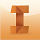## Problem description

This example shows the analysis study for a  6/4 switched reluctance motor, shown in Figure 1,  using EMS  inside Autodesk Inventor. Table 1 contains the dimensions of the studied SRM . The stator and rotor cores are both made of non-oriented M-19 silicon steel. The 4-phase windings are made of copper, and each winding has 120 turns per coil. To compute the electromagnetic torque, a parametric study was defined; in this study the torque generated by one stator phase around one rotor pole was computed. Therefore, the rotor of the switched reluctance motor was displaced to a total of 46 degrees from the unaligned position (0 deg) to the aligned position (46 deg).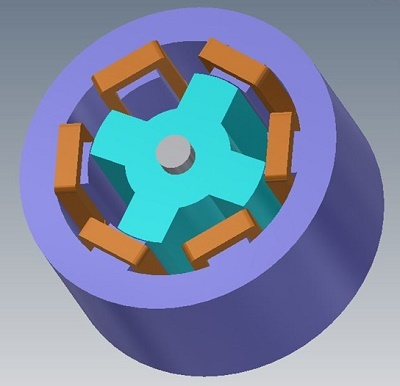Figure 1 - Switched reluctance motor

Table 1 – Main dimensions of the studied Switched reluctance motor
 Rotor poles number 4 Stator outer core diameter 72 mm Rotor outer core diameter 40.5 mm Air gap length 0.25 mm Rotor pole arc 32 deg Stator pole arc 28 deg Motor length 36 mm Shaft diameter 10 mm

## Results

Figures below show the magnetic fields generated in both rotor and stator cores at different rotor angles. Fringe and vector plots are used to illustrate the behavior of magnetic flux at various rotor positions. Figures 2a) and 3a) contain the fringe and the vector plots, respectively, at unaligned position (0 deg) of the rotor. This position is characterized by its high magnetic reluctance; at this position, the magnetic flux tries to reach the rotor cores through the two closest adjacent rotor poles. Hence, the electromagnetic torque produced at this position is almost equal to zero.

Fringe and vector plots of the magnetic fields at 18 deg rotor position are shown in Figures 2a) and 3a), respectively. At this position, the magnetic flux starts to follow closed paths from the rotor to stator poles; hence, it produces the highest torque. The fringe and vector plots results for the aligned position (46 deg) are shown in Figures 2c) and 3c), respectively. At the aligned position, the magnetic reluctance and torque values are minimal; this is because both the stator and rotor poles are in the opposite direction.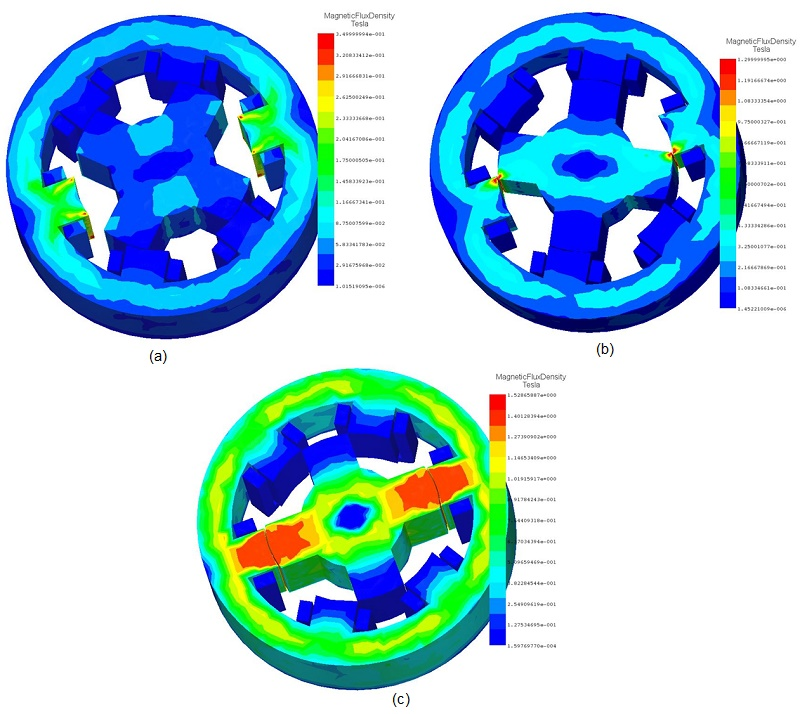Figure 2 – Fringe plots of the magnetic flux density at, a) 0 deg, b) 18 deg, c) 46 deg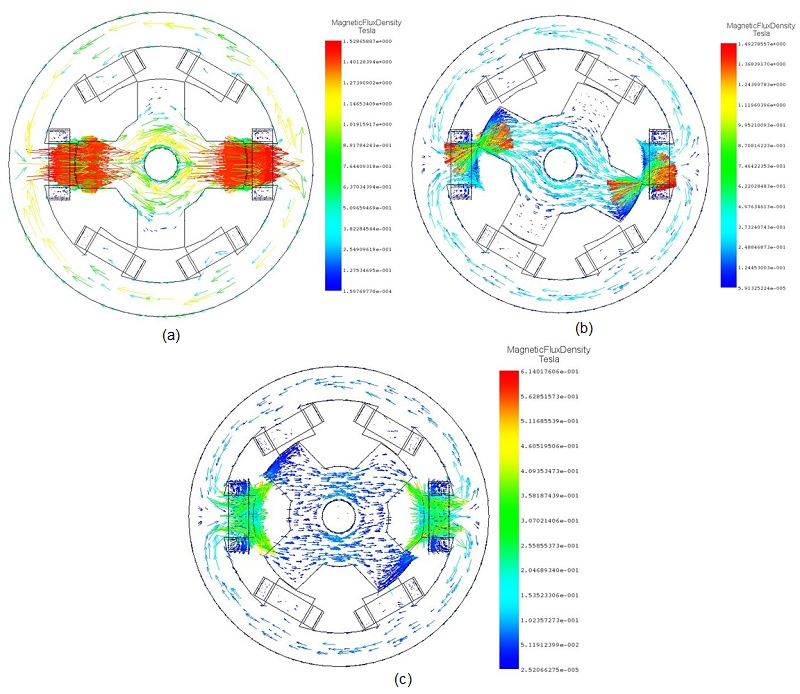Figure 3 – Vector plots of the magnetic flux density at, a) 0 deg, b) 18 deg, c) 46 deg

Figure 4 shows the torque curve versus the rotor angle. As can be seen in Figure 4, the torque rises from zero at the unaligned (0 deg), reaches its maximum value in the interval between 18 deg to 40 deg, and goes back to zero at the aligned position (46deg). The peak value of the torque is measured at measured at 18 deg and is found to be equal to 0.30 Nm (approximately).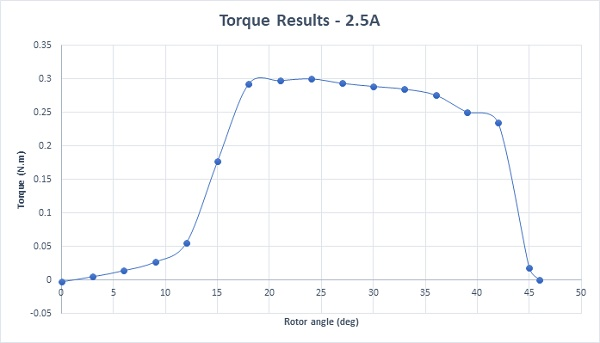Figure 4 - Static torque results – 2.5A

Since, the reluctance torque is directly proportional to the winding currents. Figure 5 shows the plots of the torque at various values of winding current.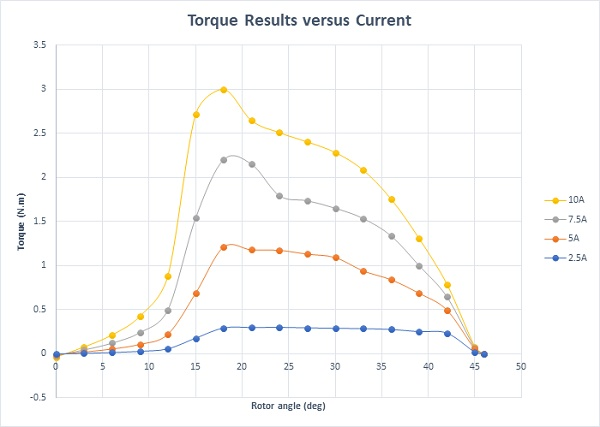Figure 5 - Static torque results versus different current rates

## Summary

EMS for Autodesk Inventor was used in this application to analyze the 6/4 switched reluctance motor. Magnetic fields and torque results were computed versus the rotor angle using a parametric study of the EMS magnetostatic module. At the end of this article, the excitation current was varied to create different torque curves versus current rates. For more application notes, please visit us at www.emworks.com.